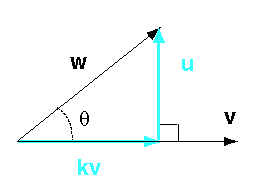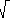(Review: ) How do you compute a unit vector?

Divide a vector by its length

# Projection ProcedureRather than memorize the formula for projection, follow what it means step-by-step. To project w onto v:

1. Compute the lengths:
| w |  =(w · w)
| v |  =(v · v)
2. Compute the unit vectors:
wu  =   w / | w |
vu  =   v / | v |
3. Compute the cosine of the angle between the vectors:
wu · vu
4. Assemble the projection:
kv  =  | w | (wu · vu) vu

### QUESTION 6:

Is w projected onto v the same vector as v projected onto w ?